Examples

Chapter 14 Class 11 Probability
Serial order wise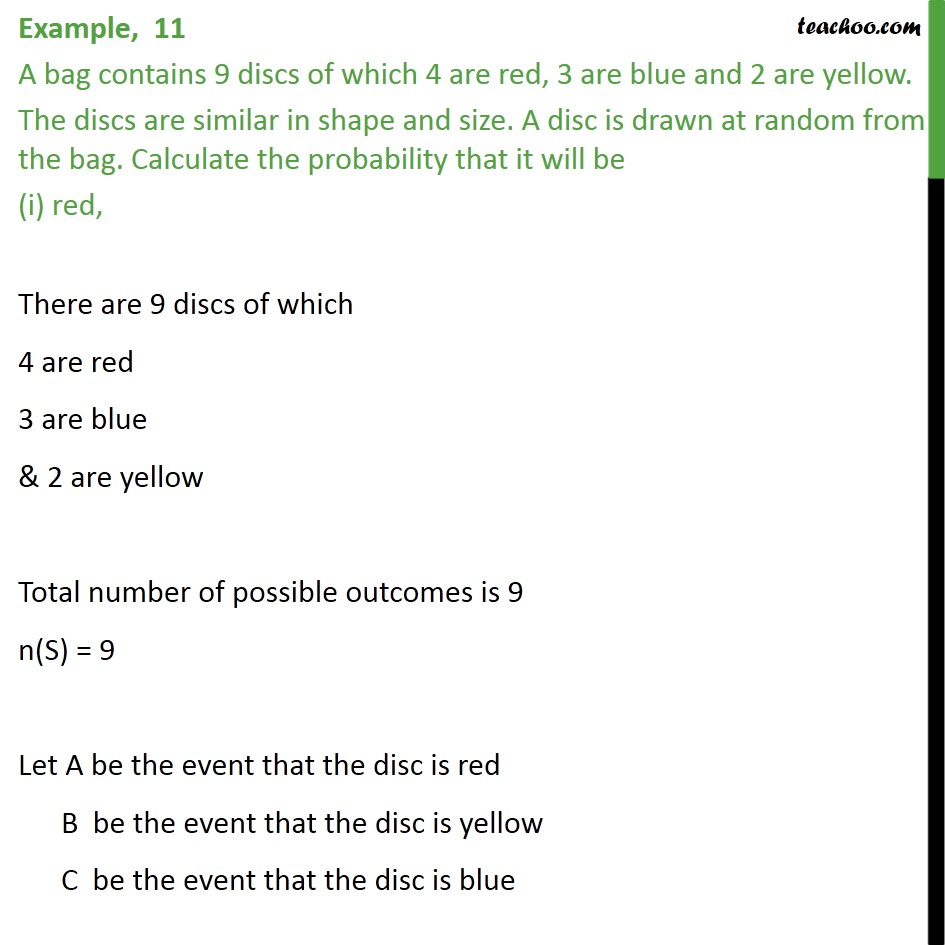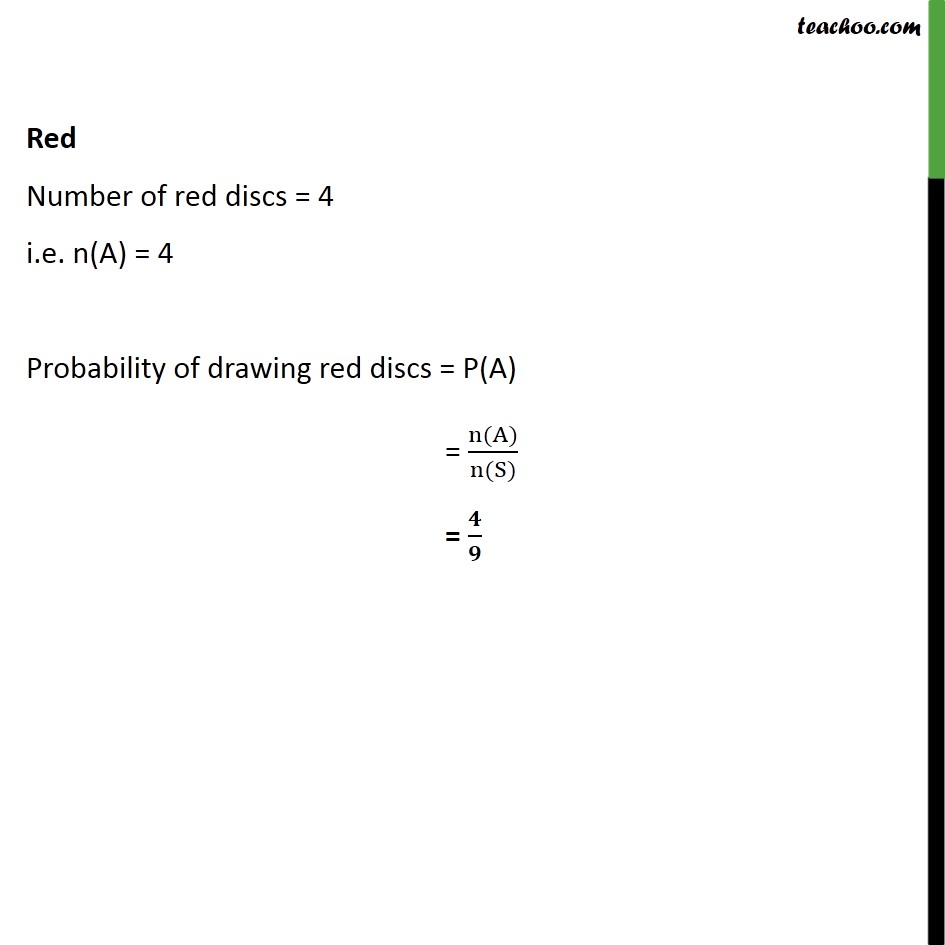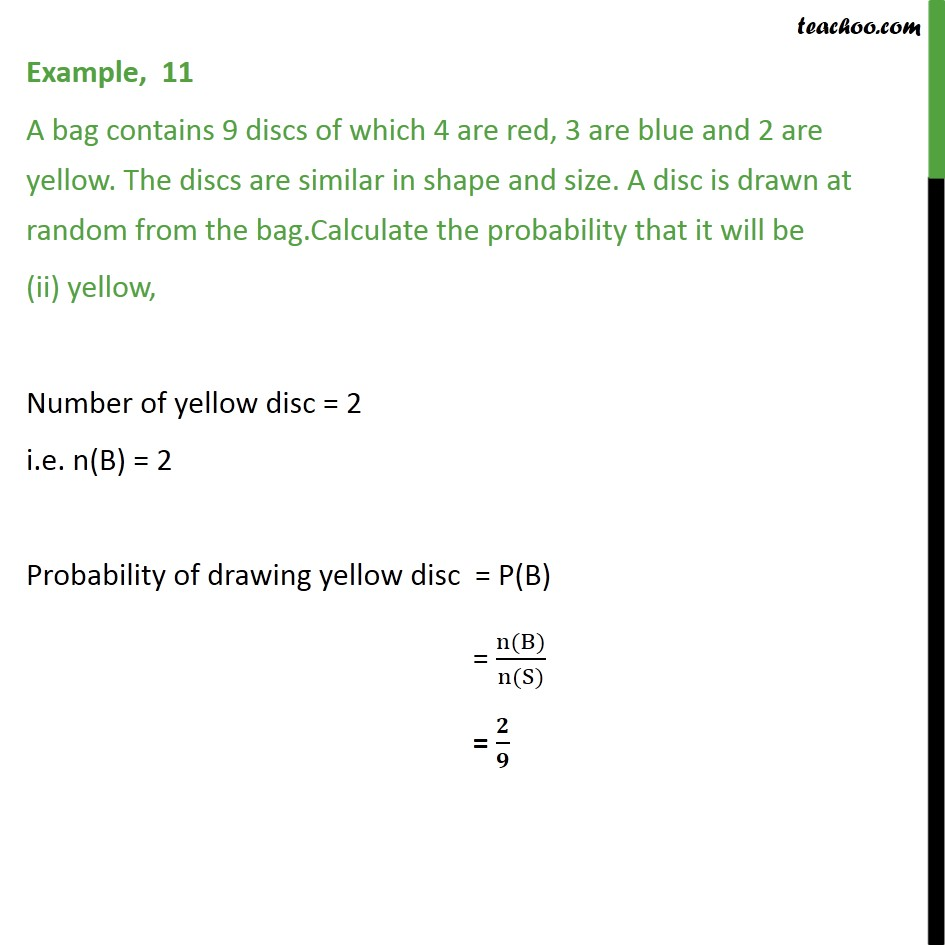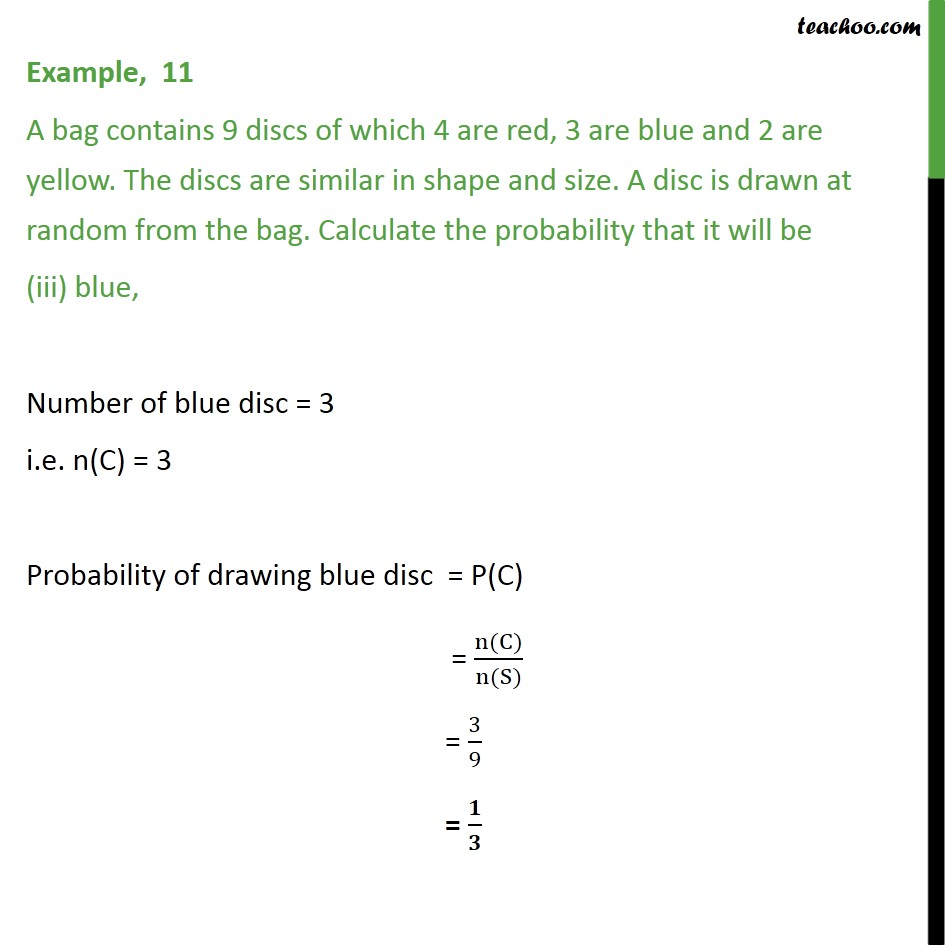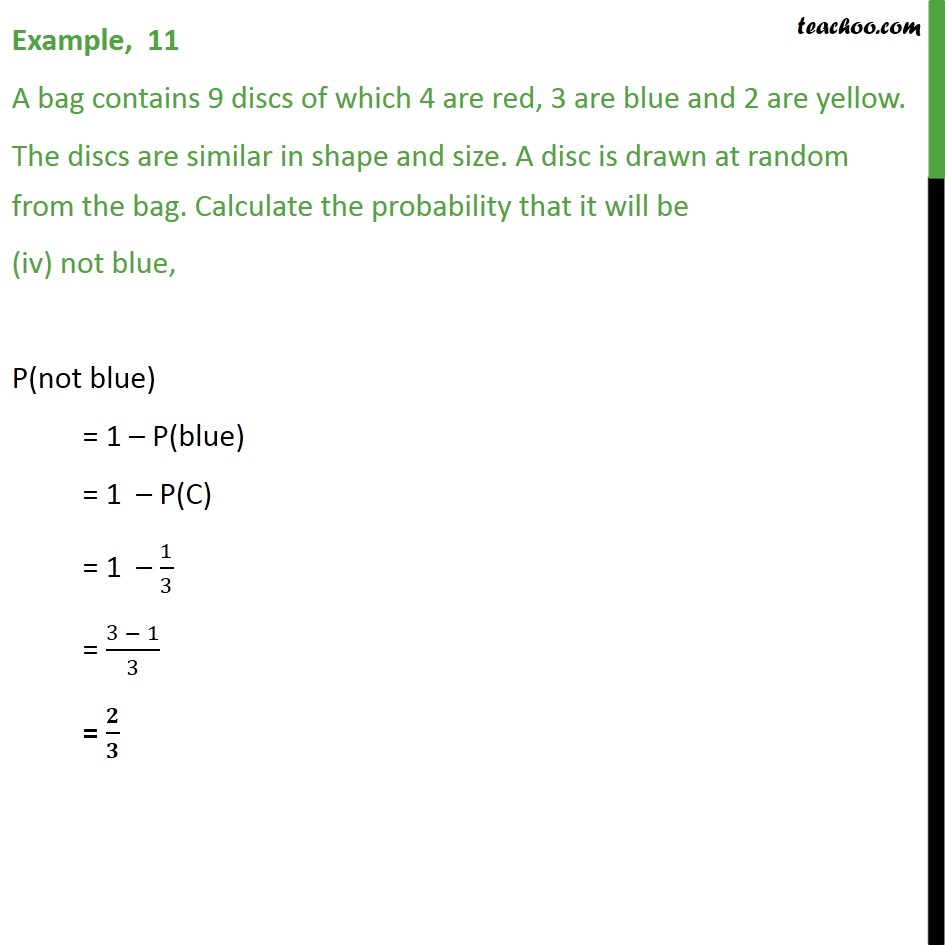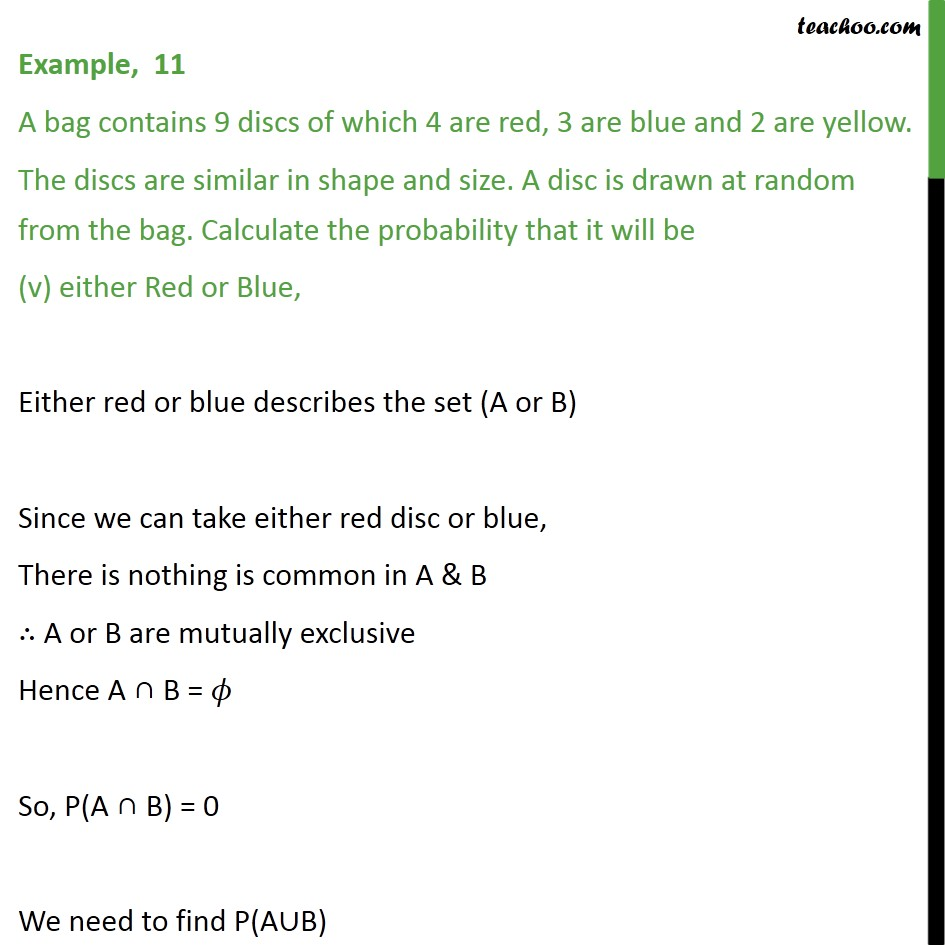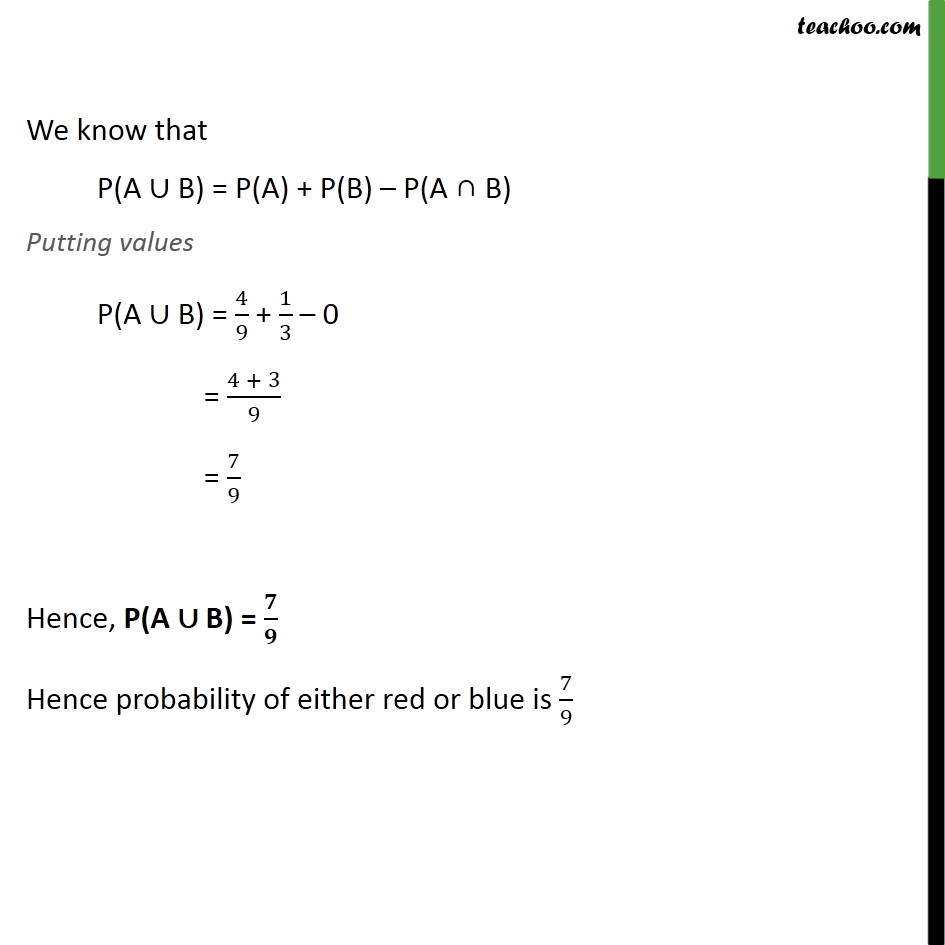Learn in your speed, with individual attention - Teachoo Maths 1-on-1 Class

### Transcript

Example, 6 A bag contains 9 discs of which 4 are red, 3 are blue and 2 are yellow. The discs are similar in shape and size. A disc is drawn at random from the bag. Calculate the probability that it will be (i) red, There are 9 discs of which 4 are red 3 are blue & 2 are yellow Total number of possible outcomes is 9 n(S) = 9 Let A be the event that the disc is red B be the event that the disc is yellow C be the event that the disc is blue Red Number of red discs = 4 i.e. n(A) = 4 Probability of drawing red discs = P(A) = n(A) n(S) = Example, 6 A bag contains 9 discs of which 4 are red, 3 are blue and 2 are yellow. The discs are similar in shape and size. A disc is drawn at random from the bag.Calculate the probability that it will be (ii) yellow, Number of yellow disc = 2 i.e. n(B) = 2 Probability of drawing yellow disc = P(B) = n(B) n(S) = Example, 6 A bag contains 9 discs of which 4 are red, 3 are blue and 2 are yellow. The discs are similar in shape and size. A disc is drawn at random from the bag. Calculate the probability that it will be (iii) blue, Number of blue disc = 3 i.e. n(C) = 3 Probability of drawing blue disc = P(C) = n(C) n(S) = 3 9 = Example, 6 A bag contains 9 discs of which 4 are red, 3 are blue and 2 are yellow. The discs are similar in shape and size. A disc is drawn at random from the bag. Calculate the probability that it will be (iv) not blue, P(not blue) = 1 P(blue) = 1 P(C) = 1 1 3 = 3 1 3 = Example, 6 A bag contains 9 discs of which 4 are red, 3 are blue and 2 are yellow. The discs are similar in shape and size. A disc is drawn at random from the bag. Calculate the probability that it will be (v) either Red or Blue, Either red or blue describes the set (A or B) Since we can take either red disc or blue, There is nothing is common in A & B A or B are mutually exclusive Hence A B = So, P(A B) = 0 We need to find P(A B) We know that P(A B) = P(A) + P(B) P(A B) Putting values P(A B) = 4 9 + 1 3 0 = 4 + 3 9 = 7 9 Hence, P(A B) = Hence probability of either red or blue is 7 9